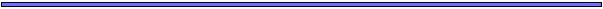## Non-Linear EquationsWe now turn our attention to models that describe the dynamics of a system in terms of NON-LINEAR equations in more than one variable.

With occasional exceptions, non-linear systems of equations do not yield general solutions (i.e. they are often intractable).

Other methods of analysing equations therefore become especially important, including

• graphical analyses
• identification of equilibria
• stability analysis of the equilibria
We will focus on two examples of non-linear systems of equations:
• Lotka-Volterra model of competition
• Spread of disease through a population## Lotka-Volterra Model of CompetitionSpecies do not exist in isolation of one another. The simple models of exponential and logistic growth fail to capture the fact that species can
• compete for resources
• assist one another
• exclude one another
• kill one another
Here we will generalize the logistic model to take into account resource competition between two species.

Model Parameters

Let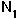= # of individuals of species 1= # of individuals of species 2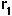= intrinsic growth rate of species 1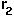= intrinsic growth rate of species 2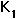= carrying capacity of species 1 when species 2 is absent= carrying capacity of species 2 when species 1 is absent## Lotka-Volterra Model of CompetitionThe assumption underlying the Lotka-Volterra competition equations is that competing species use of some of the resources available to a species (as if there were actually more individuals of the latter species):
# of individuals using resources of species 1 =+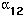is called the COMPETITION COEFFICIENT measuring the effect of an individual of species 2 on an individual of species 1. Similarly,
# of individuals using resources of species 2 =+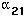Discrete Model

The assumption of the logistic model is that the number of offspring per parent decreases linearly with the number of individuals (of species 1) currently in the population.

With a second competing species also present, the number of offspring per parent depends not only on, but also on the value of:

# of offspring per parent in species 1=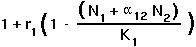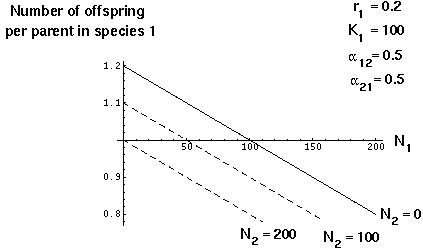## Lotka-Volterra Model of CompetitionTherefore the population size in the next generation will equal: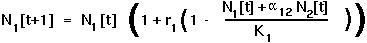Similarly,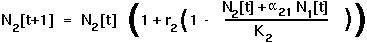Continuous Model

In this case, it is assumed that the individual's contribution to the population growth rate is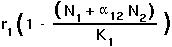for species 1for species 2

Therefore the growth rate of the entire population is equal to: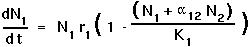for species 1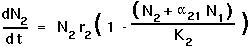for species 2

We'll focus on the discrete case in class.## Lotka-Volterra Model of CompetitionNote:

In both the discrete and continuous cases,

• Ifequals zero, then the dynamics of species 1 will follow the logistic equation we analysed before.

• Ifequals zero, then the dynamics of species 2 will follow the logistic equation we analysed before.

• Ifequals one, then individuals of species 2 compete for the resources of species 1 just as strongly as do members of species 1 (interspecific competition is as strong as intraspecific competition).

• Ifis negative, then the presence of species 2 increases the resources available to species 1.

If bothandare negative, the species are said to have a mutualistic relationship.

Iforis negative and the other is zero (or very nearly zero), the species are said to have a commensal relationship.

If one of the two is positive and one is negative, the species are said to have a parasitic relationship.

If both are positive, the species are said to have a competitive relationship.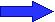We will analyse the effects of competition (with> 0 and> 0) on the dynamics of two species.## Lotka-Volterra Model of CompetitionPreliminary Graphical Analysis

The first step of an analysis might be to graph examples to see what happens to each of the species under different parameter conditions: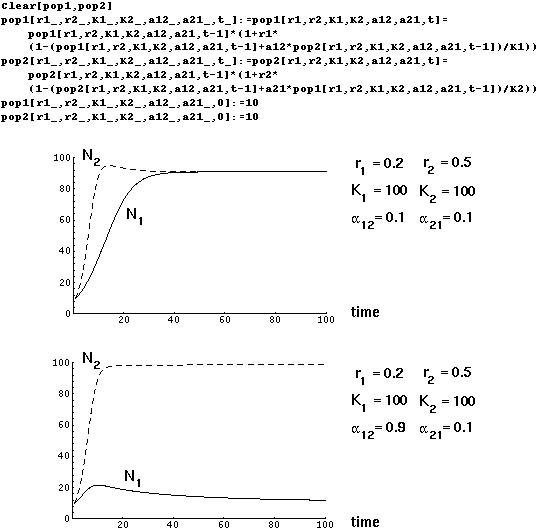Whenandare small, both species approach an equilibrium level near their carrying capactities. Ifis much higher than(species 2 impacts more strongly on the resources of species 1 than vice versa), then species 1 will be kept at low numbers by the competitive superiority of species 2.## Lotka-Volterra Model of CompetitionSuch a graphical method is only useful for specific examples, however, and doesn't give us a general picture of what will happen and when.

Identification of Equilibria

As before, we determine equilibria by finding out when the variables will stay constant over time.

Unlike the case of a single variable and a single function, however, we must find an equilibrium solution where ALL of the variables remain constant over time.

For the continuous model of competition, this requires that d/dt = 0 AND that d/dt = 0.

For the discrete model of competition, this requires that[t+1] =[t] AND that[t+1] =[t].

Setting[t+1] =[t] =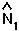in the discrete equation for species 1 gives: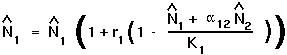There are two ways in which this equation can be satisfied: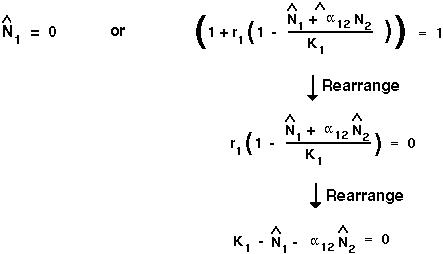## Lotka-Volterra Model of CompetitionSetting[t+1] =[t] =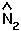in the discrete equation for species 2 gives: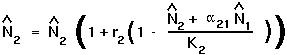Again, there are two ways in which this equation can be satisfied: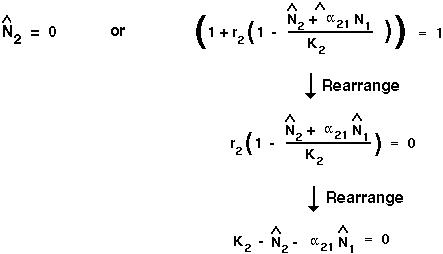At equilibrium, there are four ways to ensure that bothandremain constant over time:

• both species are extinct (= 0 and=0)
• species 1 is extinct (= 0 and=)
• species 2 is extinct (=and=0)
• both species are present.## Lotka-Volterra Model of CompetitionTo get both species to persist at equilibrium requires that both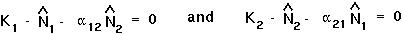To solve both equations simultaneously:

• Solve one equation forin terms of.

• Plug this value forinto the other equation.

• Solve the resulting equation forin terms of the parameters of the model.

• Finally, plug this equilibrium value forinto one of the above equations to get an equation for.Only at this point will both species be present in constant numbers over time.